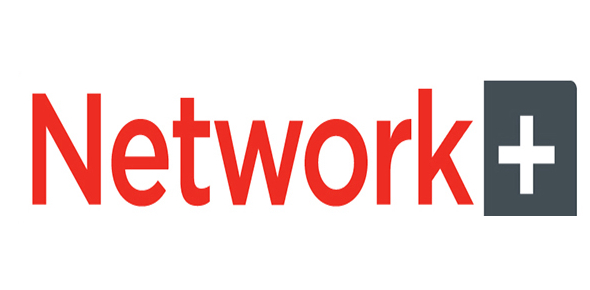# CompTIA Network+ Certification (N10-003) Part 4

30 Questions | Total Attempts: 102Settings30 CompTIA Network+ questions covering cables and connectors.

Related Topics
• 1.
What is the maximum transmission distance supported by 10GBase-ER?
• A.

300 meters

• B.

550 meters

• C.

2000 meters

• D.

10 kilometers

• E.

40 kilometers

• 2.
What is the maximum transmission distance supported by single mode 1000Base-LX?
• A.

300 meters

• B.

550 meters

• C.

2000 meters

• D.

10 kilometers

• E.

40 kilometers

• 3.
What is the maximum transmission distance supported by multimode 1000Base-LX?
• A.

300 meters

• B.

550 meters

• C.

2000 meters

• D.

10 kilometers

• E.

40 kilometers

• 4.
A site consists of two buildings that are 15 km apart.  Which of the following can you use to connect the two buildings?
• A.

100Base-FX

• B.

1000Base-LX

• C.

10GBase-ER

• D.

10GBase-LR

• 5.
What is the maximum data transmission rate supported by 10Base-2?
• A.

10 Mbps

• B.

100 Mbps

• C.

1000 Mbps

• D.

10 Gpbs

• 6.
You work with a 10Base-T network.  What is the maximum data transmission rate supported by the network?
• A.

10 Mbps

• B.

100 Mbps

• C.

1000 Mbps

• D.

10 Gbps

• 7.
You work with a Fast Ethernet network.  What is the maximum data transmission rate supported by this network?
• A.

10 Mbps

• B.

100 Mbps

• C.

1000 Mbps

• D.

10 Gbps

• 8.
What is the maximum data transmission rate supported by 100Base-FX and 100Base-TX?
• A.

10 Mbps

• B.

100 Mbps

• C.

1000 Mbps

• D.

10 Gbps

• 9.
What is the maximum data transmission rate supported by Gigabit Ethernet?
• A.

10 Mbps

• B.

100 Mbps

• C.

1000 Mbps

• D.

10 Gbps

• 10.
Your network contains a 1000Base-CX switch.  What is the maximum data transmission rate supported by this switch?
• A.

10 Mbps

• B.

100 Mbps

• C.

1000 Mbps

• D.

10 Gbps

• 11.
You work with a 1000Base-T network.  What is the maximum data transmission rate supported by the network?
• A.

10 Mbps

• B.

100 Mbps

• C.

1000 Mbps

• D.

10 Gbps

• 12.
What is the maximum data transmission rate supported by 1000Base-LX and 1000Base-SX?
• A.

10 Mbps

• B.

100 Mbps

• C.

1000 Mbps

• D.

10 Gbps

• 13.
Which of the following has a maximum data transmission rate of 10 Mbps?
• A.

10Base-2, 10Base-5 and 10Base-T

• B.

100Base-FX, 100Base-TX and FDDI

• C.

1000Base-CX, 1000Base-LX and 1000Base-SX

• D.

10 GBase-ER, 10 GBase-LR and 10 GBase-SR

• 14.
Which of the following has a maximum data transmission rate of 100 Mbps?
• A.

10Base-2, 10Base-5 and 10Base-T

• B.

100Base-FX, 100Base-TX and FDDI

• C.

1000Base-CX, 1000Base-LX and 1000Base-SX

• D.

10GBase-ER, 10GBase-LR and 10 GBase-SR

• 15.
Which of the following has a maximum data transmission rate of 1 Gbps?
• A.

10Base-2, 10Base-5 and 10Base-T

• B.

100Base-FX, 100Base-TX and FDDI

• C.

1000Base-CX, 1000Base-LX and 1000Base-SX

• D.

10GBase-ER, 10GBase-LR and 10GBase-SR

• 16.
Which of the following has a maximum data transmission rate of 10 Gbps
• A.

10Base-2, 10Base-5 and 10Base-T

• B.

100Base-FX, 100Base-TX and FDDI

• C.

1000Base-CX, 1000Base-LX and 1000Base-SX

• D.

10GBase-ER, 10GBase-LR and 10GBase-SR

• 17.
Which of the following are small form factor fiber-optic connectors? (Choose all that apply)
• A.

MT-RJ

• B.

LC

• C.

ST

• D.

RJ-45

• 18.
Which of the following is not a fiber-optic connector?
• A.

MT-RJ

• B.

LC

• C.

ST

• D.

RJ-45

• 19.
You have a 1000Base-CX switch.  Which connector does this switch use?
• A.

MT-RJ

• B.

RJ-45

• C.

HSSDC

• D.

ST

• 20.
Which type of connector would you expect to find on a 1000Base-TX network?
• A.

MT-RJ

• B.

RJ-45

• C.

HSSDC

• D.

ST

• 21.
With which of the following would you use a RJ-45 connector? (Choose all that apply)
• A.

100Base-T

• B.

1000Base-CX

• C.

1000Base-LX

• D.

1000Base-TX

• 22.
With which of the following would you use a 9-pin High Speed Serial Data Connector (HSSDC)?
• A.

Universal Serial Bus

• B.

IEEE 1394

• C.

1000Base-CX

• D.

None of the above

• 23.
Which of the following is not a fiber-optic connector?
• A.

SC

• B.

ST

• C.

MT-RJ

• D.

HSSDC

• 24.
Which of the following is not a copper connector?
• A.

HSSDC

• B.

RJ-11

• C.

RJ-45

• D.

ST

• 25.
Which of the following has two fibers?
• A.

MT-RJ

• B.

LC

• C.

SC

• D.

ST

• 26.
Which of the following has a BNC type attachment?
• A.

MT-RJ

• B.

LC

• C.

SC

• D.

ST

• 27.
Which connector does a 10Base-T hub use?
• A.

MT-RJ

• B.

RJ-45

• C.

HSSDC

• D.

ST

• 28.
How many pins would you expect to find on a connector used on a 1000Base-TX network?
• A.

4

• B.

8

• C.

9

• D.

16

• 29.
How many pins would you expect to find on a connector used on a 1000Base-CX switch?
• A.

4

• B.

8

• C.

9

• D.

16

• 30.
A RJ-45 connector has how many pins?
• A.

4

• B.

8

• C.

9

• D.

16